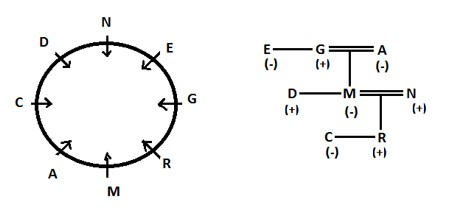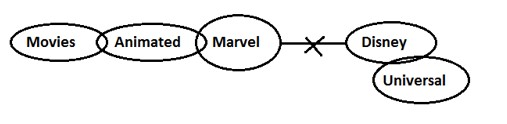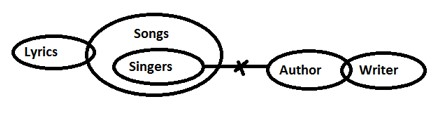# SBI PO Prelims Reasoning Ability Quiz – 14

## SBI PO Prelims 2022 Reasoning Ability Quiz

Reasoning Ability is a part of almost all major competitive exams in the country and is perhaps the most scoring section also. Aspirants who regularly practice questions have a good chance of scoring well in the Reasoning Ability Section. So here we are providing you with the SBI PO Prelims Reasoning Ability Quiz to help you prepare better. This SBI PO Prelims Reasoning Ability Quiz includes all of the most recent pattern-based questions, as well as Previous Year Questions. This SBI PO Prelims Reasoning Ability Quiz is available to you at no cost. Candidates will be provided with a detailed explanation of each question in this SBI PO Prelims Reasoning Ability Quiz. Candidates must practice this SBI PO Prelims Reasoning Ability Quiz to achieve a good score in the Reasoning Ability Section.

Directions (1-5): Study the following information carefully and answer the given questions.

There are eight members i.e. A, C, D, E, G, R, M and N in a family such that all are sitting around a circular table facing towards the centre of the table. There are two married couples in the family, which having three generations. Every male member in the family has only one sister except N. M is child of G. R sits second to the right of his maternal grandmother. Aunt of D sits third to the right of N’s wife. N sits third to the right of his son. C is the child of M and sits third to the left of her brother. D is the unmarried brother of M. E is the sister-in-law of A. N is not married to G, who is a male. Maternal Grandfather of C sits third to the left of his son who sits second to the left of his mother. E is not married.

1. How is E related to G?

(a) wife

(b) Mother

(c) Brother

(d) Sister

(e) None of these1. Who among the following sits third to the left of husband of A?

(a) Sister of G

(b) Son of G

(c) Wife of G

(d) G’s grandson

(e) R1. Who among the following is the father of C?

(a) N

(b) M

(c) D

(d) G

(e) E1. Who among the following faces N?

(a) Mother of M

(b) Father of D

(c) A

(d) C

(e) Mother of R1. Which among the following combination of persons represent the three female members of the family?

(a) M, A and R

(b) E, A and C

(c) E, A and N

(d) G, A and E

(e) None of theseDirections (6-7): In the questions given below, certain symbols are used with the following meaning:

P @ Q means P is greater than Q.

P + Q means P is either greater than or equal to Q.

P  Q means P is equal to Q.

P © Q means P is smaller than Q.

P & Q means P is either smaller than or equal to Q.

Now in each of the following questions assuming the given statements to be true, find which of the two conclusions I and II given below them is/are definitely true?

1. Statements: – A @ B, C & B, D + C, D * R

Conclusions: – I. A @ C          II. B @ D

(a) if only conclusion I is true,

(b) if only conclusion II is true,

(c) if either I or II is true,

(d) if neither I nor II is true, and

(e) if both I and II are true.1. Statements: – N * D, C & N, C @ M, R © M

Conclusions: – I. M @ D                 II. N @ R

(a) if only conclusion I is true,

(b) if only conclusion II is true,

(c) if either I or II is true,

(d) if neither I nor II is true, and

(e) if both I and II are true.Directions (8-10): In each of the questions below are given some statements followed by two conclusions numbered I and II. You have to take the given statements to be true even if, they seem to be at variance with commonly known facts. Read all the conclusions and then decide which of the given conclusions logically follows from the given statements, disregarding commonly known facts. Give answer

1. Statements: – Some Movies are Animated.

Some Animated are Marvel.

No Marvel is Disney.

Some Disney are Universal.

Conclusions: – I. All Universal being Marvel is a possibility.

II. All Marvel being universal is a possibility.

(a) If only conclusion II follows.

(b) If neither conclusion I nor conclusion II follows.

(c) If both conclusion I and conclusion II follow.

(d) If only conclusion I follows.

(e) If either conclusion I or conclusion II follows.1. Statements: – Some Lyrics are Songs.

Only Songs are singers.

No author is singer.

Some authors are writer.

Conclusions: – I. Some Writers are not singer.

II.Some singer are writer.

(a) If only conclusion II follows.

(b) If neither conclusion I nor conclusion II follows.

(c) If both conclusion I and conclusion II follow.

(d) If only conclusion I follows.

(e) If either conclusion I or conclusion II follows.1. Statements -: Some Hall are Rooms.

All Rooms are windows.

No doors are windows.

Some windows are pane.

Conclusions: – I. Some Hall are pane.

II.No Hall is Doors.

(a) If only conclusion II follows.

(b) If neither conclusion I nor conclusion II follows.

(c) If both conclusion I and conclusion II follow.

(d) If only conclusion I follows.

(e) If either conclusion I or conclusion II follows.###Click to Buy Bank MahaCombo Package

Recommended PDF’s for:

### Preparation Kit PDF

#### Most important PDF’s for Bank, SSC, Railway and Other Government Exam : Download PDF Now

AATMA-NIRBHAR Series- Static GK/Awareness Practice Ebook PDF Get PDF here
The Banking Awareness 500 MCQs E-book| Bilingual (Hindi + English) Get PDF here
AATMA-NIRBHAR Series- Banking Awareness Practice Ebook PDF Get PDF here
Computer Awareness Capsule 2.O Get PDF here
AATMA-NIRBHAR Series Quantitative Aptitude Topic-Wise PDF 2020 Get PDF here
Memory Based Puzzle E-book | 2016-19 Exams Covered Get PDF here
Caselet Data Interpretation 200 Questions Get PDF here
Puzzle & Seating Arrangement E-Book for BANK PO MAINS (Vol-1) Get PDF here

3